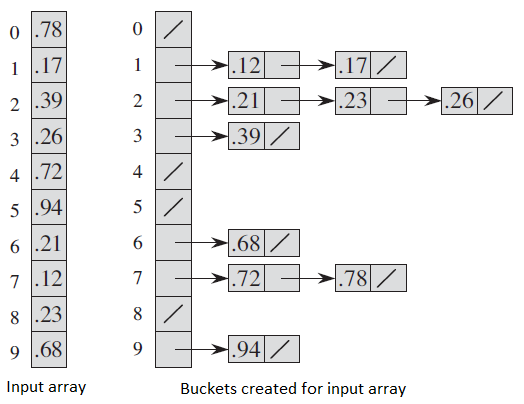# Bucket Sort Basic Information

suggest change

Bucket Sort is a sorting algorithm in which elements of input array are distributed in buckets. After distributing all the elements, buckets are sorted individually by another sorting algorithm. Sometimes it is also sorted by recursive method.

Pseudo code for Bucket Sort

1. Let n be the length of the input list L;
2. For each element i from L
3. If B[i] is not empty
4. Put A[i] into B[i];
5. Else B[i] := A[i]
6. return Concat B[i .. n] into one sorted list;

Example of bucket sort:Mostly people uses insertion paradigm for little bit of optimization. Auxiliary Space: `O{n}`